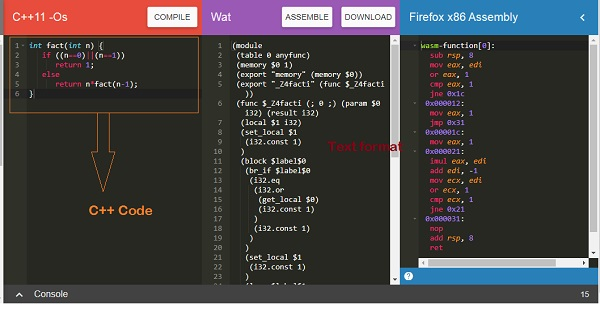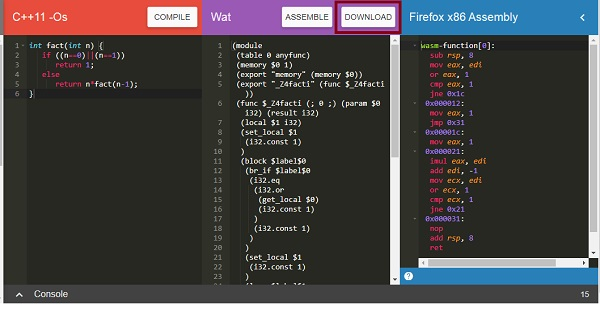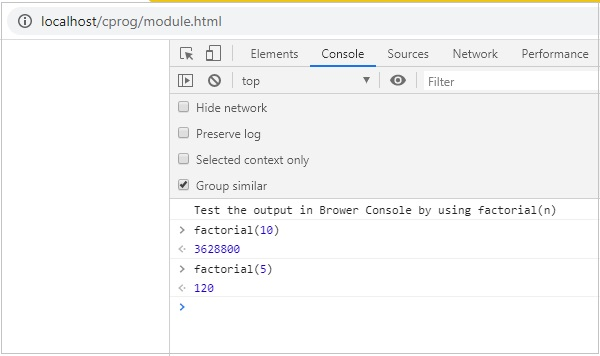# WebAssembly - Modules

We have seen how to get a .wasm file from c /c++ code. In this chapter, we will convert the wasm into a WebAssembly module and execute the same in the browser.

Let us use the C++ Factorial code as shown below −

```int fact(int n) {
if ((n==0)||(n==1))
return 1;
else
return n*fact(n-1);
}
```

Open Wasm Explorer which is available at https://mbebenita.github.io/WasmExplorer/ as shown below −The first column has the C++ factorial function, the 2nd column has the WebAssembly text format and the last column has x86 Assembly code.

The WebAssembly Text format −

```(module
(table 0 anyfunc)
(memory \$0 1)
(export "memory" (memory \$0))
(export "_Z4facti" (func \$_Z4facti))
(func \$_Z4facti (; 0 ;) (param \$0 i32) (result i32)
(local \$1 i32)
(set_local \$1
(i32.const 1)
)
(block \$label\$0
(br_if \$label\$0
(i32.eq
(i32.or
(get_local \$0)
(i32.const 1)
)
(i32.const 1)
)
)
(set_local \$1
(i32.const 1)
)
(loop \$label\$1
(set_local \$1
(i32.mul
(get_local \$0)
(get_local \$1)
)
)
(br_if \$label\$1
(i32.ne
(i32.or
(tee_local \$0
(get_local \$0)
(i32.const -1)
)
)
(i32.const 1)
)
(i32.const 1)
)
)
)
)
(get_local \$1)
)
)
```

The C++ function fact has been exported as “_Z4facti” in WebAssembly Text format.Now to convert the .wasm code to the module we have to do the following −

## Step 1

Convert the .wasm into arraybuffer by using ArrayBuffer. The ArrayBuffer object will return you a fixed-length binary data buffer.

## Step 2

The bytes from ArrayBuffer have to be compiled into a module by using WebAssembly.compile(buffer) function.

The WebAssembly.compile() function compiles and returns a WebAssembly.Module from the bytes given.

Here, is the Javascript code that is discussed in Step 1 and 2.

```<script type="text/javascript">
let factorial;
fetch("factorial.wasm")
.then(bytes => bytes.arrayBuffer())
.then(mod => WebAssembly.compile(mod))
.then(module => {return new WebAssembly.Instance(module) })
.then(instance => {

factorial = instance.exports._Z4facti;
console.log('Test the output in Brower Console by using factorial(n)');
});
</script>
```

## Code Explanation

• Javascript browser API fetch is used to get the contents of factorial.wasm.

• The content is converted to bytes using arrayBuffer().

• The module is created from bytes by calling WebAssembly.compile(mod).

• The instance of a module is created using new

WebAssembly.Instance(module)

• The factorial function export _Z4facti is assigned to variable factorial by using WebAssembly.Module.exports().

## Example

Here, is the module.html along with the javascript code −

module.html

```<!doctype html>
<html>
<meta charset="utf-8">
<title>WebAssembly Module</title>
<body>
<script>
let factorial;
fetch("factorial.wasm")
.then(bytes => bytes.arrayBuffer())
.then(mod => WebAssembly.compile(mod))
.then(module => {return new WebAssembly.Instance(module) })
.then(instance => {
factorial = instance.exports._Z4facti;
console.log('Test the output in Browser Console by using factorial(n)');
});
</script>
</body>
</html>
```

## Output

Execute module.html in the browser to see the output −